AVL Tree

# AVL Tree

#### In this tutorial, you will learn what an avl tree is. Also, you will find working examples of various operations performed on an avl tree in C, C++, Java and Python.

AVL tree is a self-balancing binary search tree in which each node maintains extra information called a balance factor whose value is either -1, 0 or +1.

AVL tree got its name after its inventor Georgy Adelson-Velsky and Landis.

## Balance Factor

Balance factor of a node in an AVL tree is the difference between the height of the left subtree and that of the right subtree of that node.

Balance Factor = (Height of Left Subtree - Height of Right Subtree) or (Height of Right Subtree - Height of Left Subtree)

The self balancing property of an avl tree is maintained by the balance factor. The value of balance factor should always be -1, 0 or +1.

An example of a balanced avl tree is:

## Operations on an AVL tree

Various operations that can be performed on an AVL tree are:

## Rotating the subtrees in an AVL Tree

In rotation operation, the positions of the nodes of a subtree are interchanged.

There are two types of rotations:

### Left Rotate

In left-rotation, the arrangement of the nodes on the right is transformed into the arrangements on the left node.

Algorithm

2. If y has a left subtree, assign x as the parent of the left subtree of y.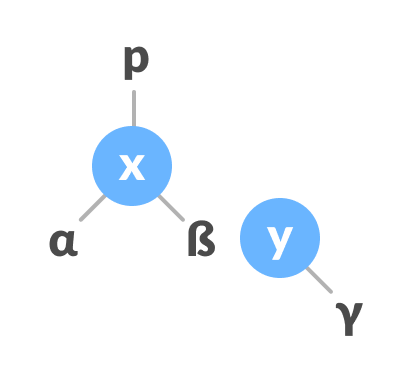Assign x as the parent of the left subtree of y
3. If the parent of x is `NULL`, make y as the root of the tree.
4. Else if x is the left child of p, make y as the left child of p.
5. Else assign y as the right child of p.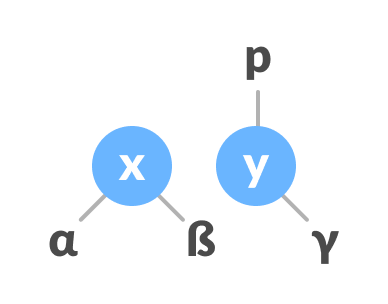Change the parent of x to that of y
6. Make y as the parent of x.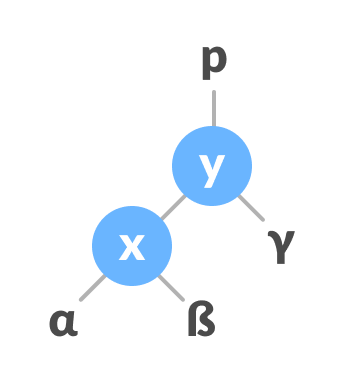Assign y as the parent of x.

### Right Rotate

In left-rotation, the arrangement of the nodes on the left is transformed into the arrangements on the right node.

2. If x has a right subtree, assign y as the parent of the right subtree of x.Assign y as the parent of the right subtree of x
3. If the parent of y is `NULL`, make x as the root of the tree.
4. Else if y is the right child of its parent p, make x as the right child of p.
5. Else assign x as the left child of p.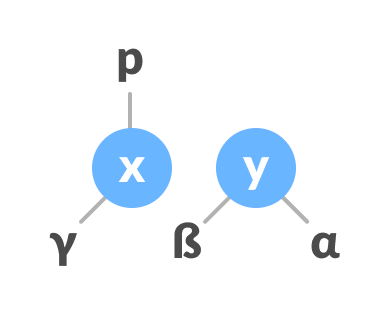Assign the parent of y as the parent of x.
6. Make x as the parent of y.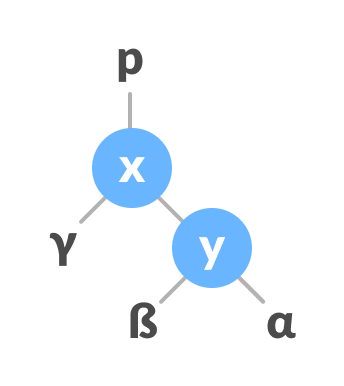Assign x as the parent of y

### Left-Right and Right-Left Rotate

In left-right rotation, the arrangements are first shifted to the left and then to the right.

In right-left rotation, the arrangements are first shifted to the right and then to the left.

## Algorithm to insert a newNode

A newNode is always inserted as a leaf node with balance factor equal to 0.

2. Go to the appropriate leaf node to insert a newNode using the following recursive steps. Compare newKey with rootKey of the current tree.
1. If newKey < rootKey, call insertion algorithm on the left subtree of the current node until the leaf node is reached.
2. Else if newKey > rootKey, call insertion algorithm on the right subtree of current node until the leaf node is reached.
3. Compare leafKey obtained from the above steps with newKey:
1. If newKey < leafKey, make newNode as the leftChild of leafNode.
2. Else, make newNode as rightChild of leafNode.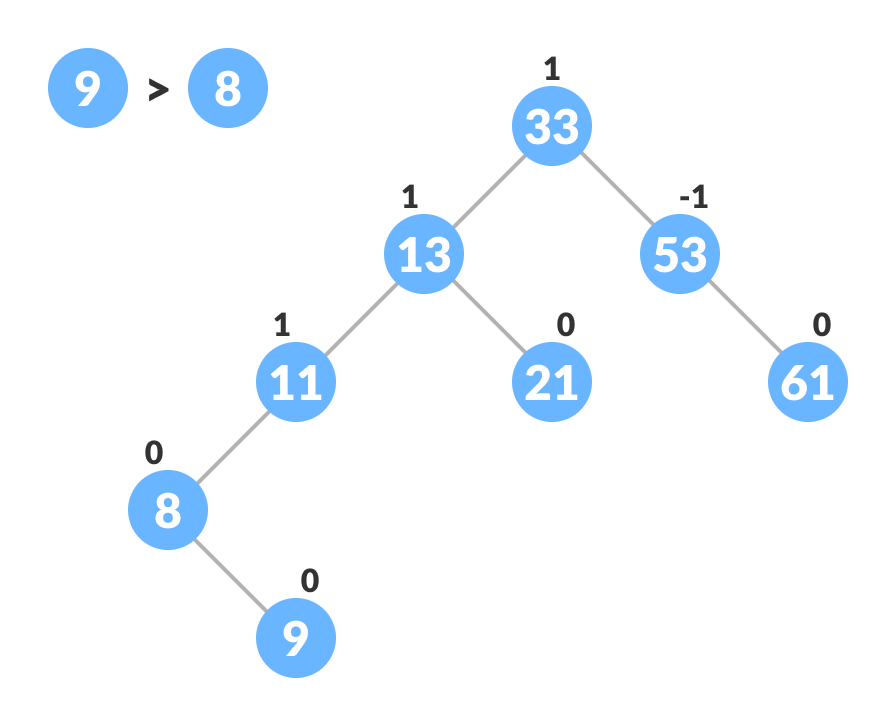Inserting the new node
4. Update balanceFactor of the nodes.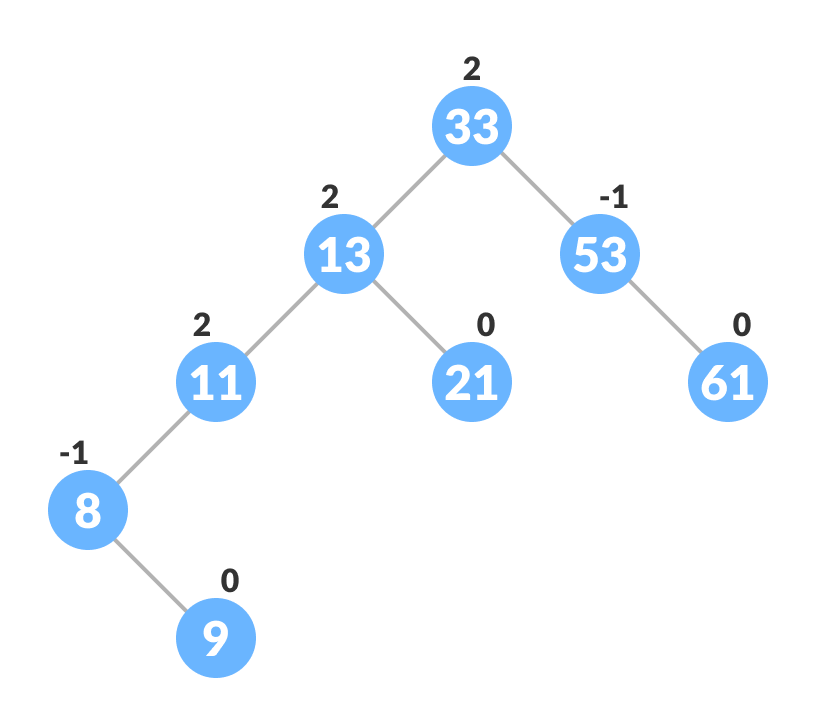Updating the balance factor after insertion
5. If the nodes are unbalanced, then rebalance the node.
1. If balanceFactor > 1, it means the height of the left subtree is greater than that of the right subtree. So, do a right rotation or left-right rotation
1. If newNodeKey < leftChildKey do right rotation.
2. If balanceFactor < -1, it means the height of the right subtree is greater than that of the left subtree. So, do right rotation or right-left rotation
1. If newNodeKey > rightChildKey do left rotation.
2. Else, do right-left rotation

## Algorithm to Delete a node

A node is always deleted as a leaf node. After deleting a node, the balance factors of the nodes get changed. In order to rebalance the balance factor, suitable rotations are performed.

1. Locate nodeToBeDeleted (recursion is used to find nodeToBeDeleted in the code used below).Locating the node to be deleted
2. There are three cases for deleting a node:
1. If nodeToBeDeleted is the leaf node (ie. does not have any child), then remove nodeToBeDeleted.
2. If nodeToBeDeleted has one child, then substitute the contents of nodeToBeDeleted with that of the child. Remove the child.
3. If nodeToBeDeleted has two children, find the inorder successor w of nodeToBeDeleted (ie. node with a minimum value of key in the right subtree).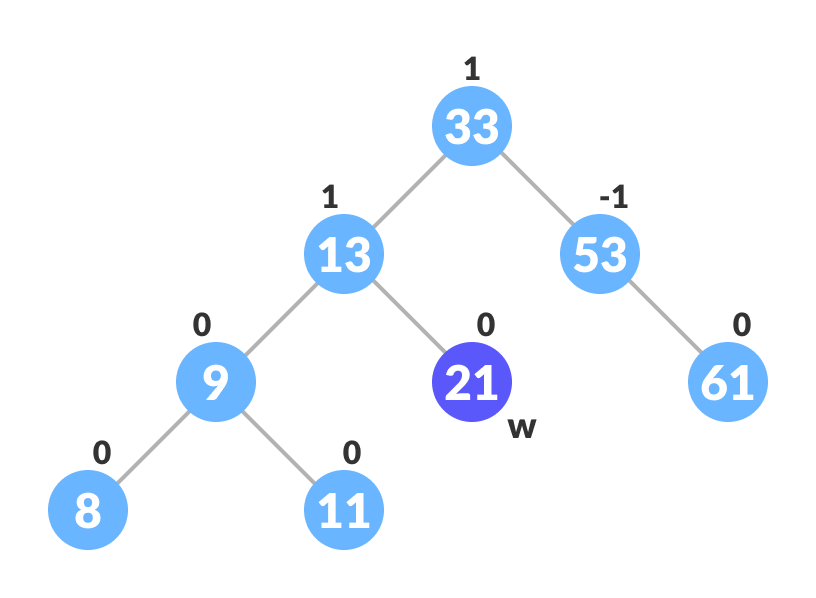Finding the successor
1. Substitute the contents of nodeToBeDeleted with that of w.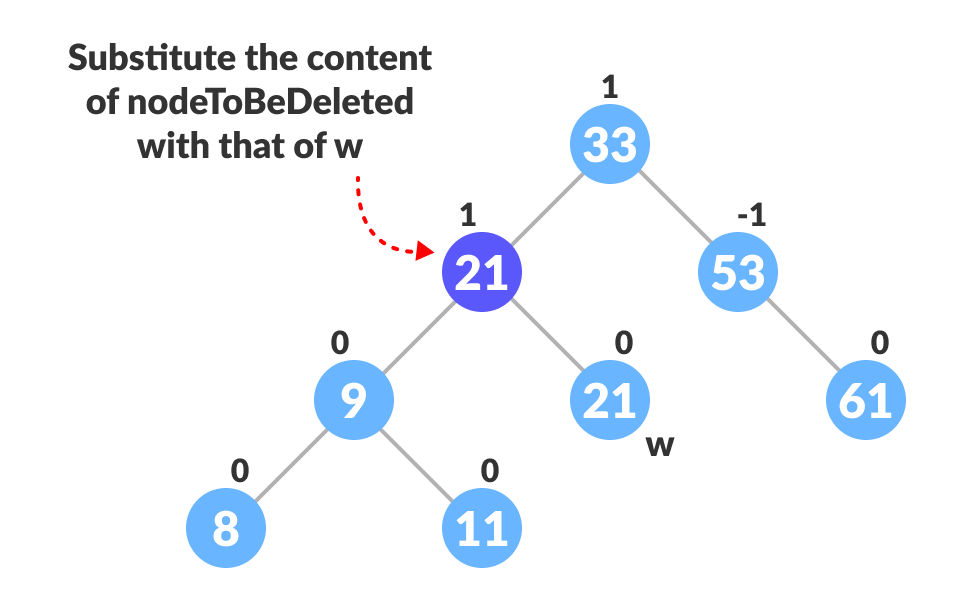Substitute the node to be deleted
4. Rebalance the tree if the balance factor of any of the nodes is not equal to -1, 0 or 1.
1. If balanceFactor of currentNode > 1,
1. If balanceFactor of leftChild >= 0, do right rotation.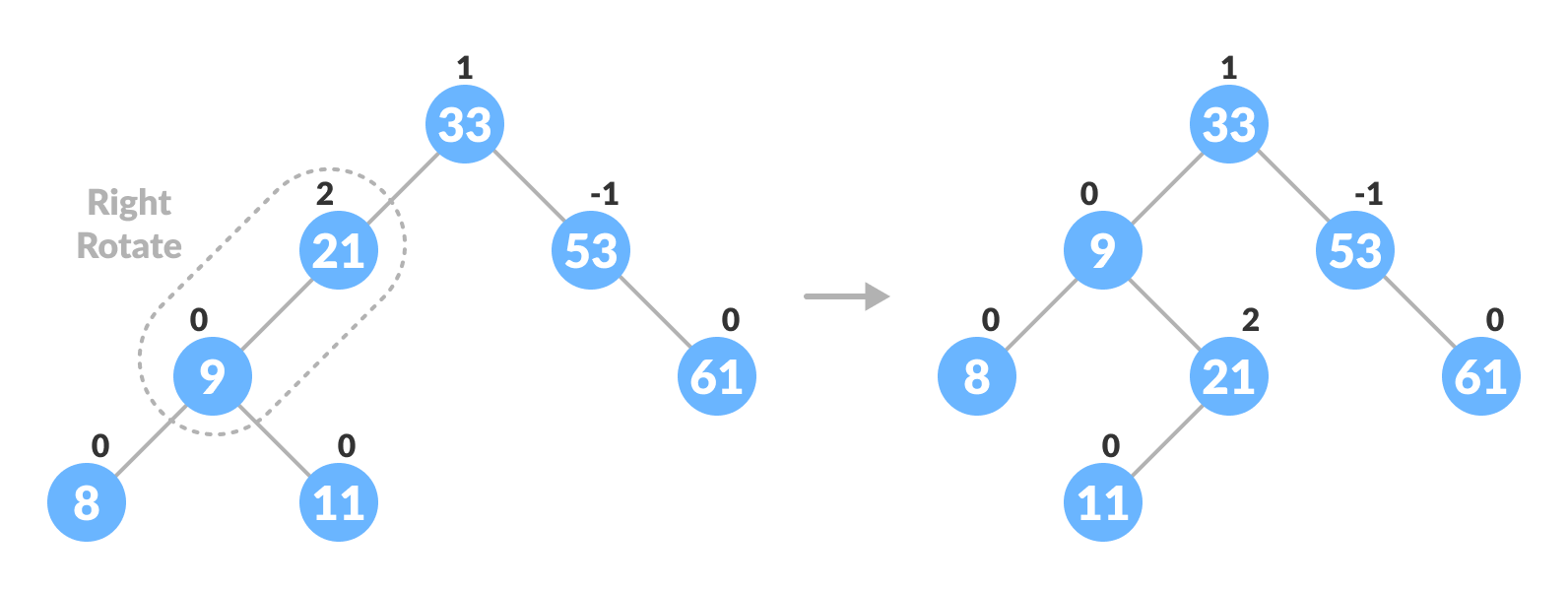Right-rotate for balancing the tree
2. Else do left-right rotation.
2. If balanceFactor of currentNode < -1,
1. If balanceFactor of rightChild <= 0, do left rotation.
2. Else do right-left rotation.

## Python, Java and C/C++ Examples

``````# AVL tree implementation in Python

import sys

# Create a tree node
class TreeNode(object):
def __init__(self, key):
self.key = key
self.left = None
self.right = None
self.height = 1

class AVLTree(object):

# Function to insert a node
def insert_node(self, root, key):

# Find the correct location and insert the node
if not root:
return TreeNode(key)
elif key < root.key:
root.left = self.insert_node(root.left, key)
else:
root.right = self.insert_node(root.right, key)

root.height = 1 + max(self.getHeight(root.left),
self.getHeight(root.right))

# Update the balance factor and balance the tree
balanceFactor = self.getBalance(root)
if balanceFactor > 1:
if key < root.left.key:
return self.rightRotate(root)
else:
root.left = self.leftRotate(root.left)
return self.rightRotate(root)

if balanceFactor < -1:
if key > root.right.key:
return self.leftRotate(root)
else:
root.right = self.rightRotate(root.right)
return self.leftRotate(root)

return root

# Function to delete a node
def delete_node(self, root, key):

# Find the node to be deleted and remove it
if not root:
return root
elif key < root.key:
root.left = self.delete_node(root.left, key)
elif key > root.key:
root.right = self.delete_node(root.right, key)
else:
if root.left is None:
temp = root.right
root = None
return temp
elif root.right is None:
temp = root.left
root = None
return temp
temp = self.getMinValueNode(root.right)
root.key = temp.key
root.right = self.delete_node(root.right,
temp.key)
if root is None:
return root

# Update the balance factor of nodes
root.height = 1 + max(self.getHeight(root.left),
self.getHeight(root.right))

balanceFactor = self.getBalance(root)

# Balance the tree
if balanceFactor > 1:
if self.getBalance(root.left) >= 0:
return self.rightRotate(root)
else:
root.left = self.leftRotate(root.left)
return self.rightRotate(root)
if balanceFactor < -1:
if self.getBalance(root.right) <= 0:
return self.leftRotate(root)
else:
root.right = self.rightRotate(root.right)
return self.leftRotate(root)
return root

# Function to perform left rotation
def leftRotate(self, z):
y = z.right
T2 = y.left
y.left = z
z.right = T2
z.height = 1 + max(self.getHeight(z.left),
self.getHeight(z.right))
y.height = 1 + max(self.getHeight(y.left),
self.getHeight(y.right))
return y

# Function to perform right rotation
def rightRotate(self, z):
y = z.left
T3 = y.right
y.right = z
z.left = T3
z.height = 1 + max(self.getHeight(z.left),
self.getHeight(z.right))
y.height = 1 + max(self.getHeight(y.left),
self.getHeight(y.right))
return y

# Get the height of the node
def getHeight(self, root):
if not root:
return 0
return root.height

# Get balance factore of the node
def getBalance(self, root):
if not root:
return 0
return self.getHeight(root.left) - self.getHeight(root.right)

def getMinValueNode(self, root):
if root is None or root.left is None:
return root
return self.getMinValueNode(root.left)

def preOrder(self, root):
if not root:
return
print("{0} ".format(root.key), end="")
self.preOrder(root.left)
self.preOrder(root.right)

# Print the tree
def printHelper(self, currPtr, indent, last):
if currPtr != None:
sys.stdout.write(indent)
if last:
sys.stdout.write("R----")
indent += "     "
else:
sys.stdout.write("L----")
indent += "|    "
print(currPtr.key)
self.printHelper(currPtr.left, indent, False)
self.printHelper(currPtr.right, indent, True)

myTree = AVLTree()
root = None
nums = [33, 13, 52, 9, 21, 61, 8, 11]
for num in nums:
root = myTree.insert_node(root, num)
myTree.printHelper(root, "", True)
key = 13
root = myTree.delete_node(root, key)
print("After Deletion: ")
myTree.printHelper(root, "", True)``````
``````// AVL tree implementation in Java

// Create node
class Node {
int item, height;
Node left, right;

Node(int d) {
item = d;
height = 1;
}
}

// Tree class
class AVLTree {
Node root;

int height(Node N) {
if (N == null)
return 0;
return N.height;
}

int max(int a, int b) {
return (a > b) ? a : b;
}

Node rightRotate(Node y) {
Node x = y.left;
Node T2 = x.right;
x.right = y;
y.left = T2;
y.height = max(height(y.left), height(y.right)) + 1;
x.height = max(height(x.left), height(x.right)) + 1;
return x;
}

Node leftRotate(Node x) {
Node y = x.right;
Node T2 = y.left;
y.left = x;
x.right = T2;
x.height = max(height(x.left), height(x.right)) + 1;
y.height = max(height(y.left), height(y.right)) + 1;
return y;
}

// Get balance factor of a node
int getBalanceFactor(Node N) {
if (N == null)
return 0;
return height(N.left) - height(N.right);
}

// Insert a node
Node insertNode(Node node, int item) {

// Find the position and insert the node
if (node == null)
return (new Node(item));
if (item < node.item)
node.left = insertNode(node.left, item);
else if (item > node.item)
node.right = insertNode(node.right, item);
else
return node;

// Update the balance factor of each node
// And, balance the tree
node.height = 1 + max(height(node.left), height(node.right));
int balanceFactor = getBalanceFactor(node);
if (balanceFactor > 1) {
if (item < node.left.item) {
return rightRotate(node);
} else if (item > node.left.item) {
node.left = leftRotate(node.left);
return rightRotate(node);
}
}
if (balanceFactor < -1) {
if (item > node.right.item) {
return leftRotate(node);
} else if (item < node.right.item) {
node.right = rightRotate(node.right);
return leftRotate(node);
}
}
return node;
}

Node nodeWithMimumValue(Node node) {
Node current = node;
while (current.left != null)
current = current.left;
return current;
}

// Delete a node
Node deleteNode(Node root, int item) {

// Find the node to be deleted and remove it
if (root == null)
return root;
if (item < root.item)
root.left = deleteNode(root.left, item);
else if (item > root.item)
root.right = deleteNode(root.right, item);
else {
if ((root.left == null) || (root.right == null)) {
Node temp = null;
if (temp == root.left)
temp = root.right;
else
temp = root.left;
if (temp == null) {
temp = root;
root = null;
} else
root = temp;
} else {
Node temp = nodeWithMimumValue(root.right);
root.item = temp.item;
root.right = deleteNode(root.right, temp.item);
}
}
if (root == null)
return root;

// Update the balance factor of each node and balance the tree
root.height = max(height(root.left), height(root.right)) + 1;
int balanceFactor = getBalanceFactor(root);
if (balanceFactor > 1) {
if (getBalanceFactor(root.left) >= 0) {
return rightRotate(root);
} else {
root.left = leftRotate(root.left);
return rightRotate(root);
}
}
if (balanceFactor < -1) {
if (getBalanceFactor(root.right) <= 0) {
return leftRotate(root);
} else {
root.right = rightRotate(root.right);
return leftRotate(root);
}
}
return root;
}

void preOrder(Node node) {
if (node != null) {
System.out.print(node.item + " ");
preOrder(node.left);
preOrder(node.right);
}
}

// Print the tree
private void printTree(Node currPtr, String indent, boolean last) {
if (currPtr != null) {
System.out.print(indent);
if (last) {
System.out.print("R----");
indent += "   ";
} else {
System.out.print("L----");
indent += "|  ";
}
System.out.println(currPtr.item);
printTree(currPtr.left, indent, false);
printTree(currPtr.right, indent, true);
}
}

// Driver code
public static void main(String[] args) {
AVLTree tree = new AVLTree();
tree.root = tree.insertNode(tree.root, 33);
tree.root = tree.insertNode(tree.root, 13);
tree.root = tree.insertNode(tree.root, 53);
tree.root = tree.insertNode(tree.root, 9);
tree.root = tree.insertNode(tree.root, 21);
tree.root = tree.insertNode(tree.root, 61);
tree.root = tree.insertNode(tree.root, 8);
tree.root = tree.insertNode(tree.root, 11);
tree.printTree(tree.root, "", true);
tree.root = tree.deleteNode(tree.root, 13);
System.out.println("After Deletion: ");
tree.printTree(tree.root, "", true);
}
}``````
``````// AVL tree implementation in C

#include <stdio.h>
#include <stdlib.h>

// Create Node
struct Node {
int key;
struct Node *left;
struct Node *right;
int height;
};

int max(int a, int b);

// Calculate height
int height(struct Node *N) {
if (N == NULL)
return 0;
return N->height;
}

int max(int a, int b) {
return (a > b) ? a : b;
}

// Create a node
struct Node *newNode(int key) {
struct Node *node = (struct Node *)
malloc(sizeof(struct Node));
node->key = key;
node->left = NULL;
node->right = NULL;
node->height = 1;
return (node);
}

// Right rotate
struct Node *rightRotate(struct Node *y) {
struct Node *x = y->left;
struct Node *T2 = x->right;

x->right = y;
y->left = T2;

y->height = max(height(y->left), height(y->right)) + 1;
x->height = max(height(x->left), height(x->right)) + 1;

return x;
}

// Left rotate
struct Node *leftRotate(struct Node *x) {
struct Node *y = x->right;
struct Node *T2 = y->left;

y->left = x;
x->right = T2;

x->height = max(height(x->left), height(x->right)) + 1;
y->height = max(height(y->left), height(y->right)) + 1;

return y;
}

// Get the balance factor
int getBalance(struct Node *N) {
if (N == NULL)
return 0;
return height(N->left) - height(N->right);
}

// Insert node
struct Node *insertNode(struct Node *node, int key) {
// Find the correct position to insertNode the node and insertNode it
if (node == NULL)
return (newNode(key));

if (key < node->key)
node->left = insertNode(node->left, key);
else if (key > node->key)
node->right = insertNode(node->right, key);
else
return node;

// Update the balance factor of each node and
// Balance the tree
node->height = 1 + max(height(node->left),
height(node->right));

int balance = getBalance(node);
if (balance > 1 && key < node->left->key)
return rightRotate(node);

if (balance < -1 && key > node->right->key)
return leftRotate(node);

if (balance > 1 && key > node->left->key) {
node->left = leftRotate(node->left);
return rightRotate(node);
}

if (balance < -1 && key < node->right->key) {
node->right = rightRotate(node->right);
return leftRotate(node);
}

return node;
}

struct Node *minValueNode(struct Node *node) {
struct Node *current = node;

while (current->left != NULL)
current = current->left;

return current;
}

// Delete a nodes
struct Node *deleteNode(struct Node *root, int key) {
// Find the node and delete it
if (root == NULL)
return root;

if (key < root->key)
root->left = deleteNode(root->left, key);

else if (key > root->key)
root->right = deleteNode(root->right, key);

else {
if ((root->left == NULL) || (root->right == NULL)) {
struct Node *temp = root->left ? root->left : root->right;

if (temp == NULL) {
temp = root;
root = NULL;
} else
*root = *temp;
free(temp);
} else {
struct Node *temp = minValueNode(root->right);

root->key = temp->key;

root->right = deleteNode(root->right, temp->key);
}
}

if (root == NULL)
return root;

// Update the balance factor of each node and
// balance the tree
root->height = 1 + max(height(root->left),
height(root->right));

int balance = getBalance(root);
if (balance > 1 && getBalance(root->left) >= 0)
return rightRotate(root);

if (balance > 1 && getBalance(root->left) < 0) {
root->left = leftRotate(root->left);
return rightRotate(root);
}

if (balance < -1 && getBalance(root->right) <= 0)
return leftRotate(root);

if (balance < -1 && getBalance(root->right) > 0) {
root->right = rightRotate(root->right);
return leftRotate(root);
}

return root;
}

// Print the tree
void printPreOrder(struct Node *root) {
if (root != NULL) {
printf("%d ", root->key);
printPreOrder(root->left);
printPreOrder(root->right);
}
}

int main() {
struct Node *root = NULL;

root = insertNode(root, 2);
root = insertNode(root, 1);
root = insertNode(root, 7);
root = insertNode(root, 4);
root = insertNode(root, 5);
root = insertNode(root, 3);
root = insertNode(root, 8);

printPreOrder(root);

root = deleteNode(root, 3);

printf("\nAfter deletion: ");
printPreOrder(root);

return 0;
}``````
``````// AVL tree implementation in C++

#include <iostream>
using namespace std;

class Node {
public:
int key;
Node *left;
Node *right;
int height;
};

int max(int a, int b);

// Calculate height
int height(Node *N) {
if (N == NULL)
return 0;
return N->height;
}

int max(int a, int b) {
return (a > b) ? a : b;
}

// New node creation
Node *newNode(int key) {
Node *node = new Node();
node->key = key;
node->left = NULL;
node->right = NULL;
node->height = 1;
return (node);
}

// Rotate right
Node *rightRotate(Node *y) {
Node *x = y->left;
Node *T2 = x->right;
x->right = y;
y->left = T2;
y->height = max(height(y->left),
height(y->right)) +
1;
x->height = max(height(x->left),
height(x->right)) +
1;
return x;
}

// Rotate left
Node *leftRotate(Node *x) {
Node *y = x->right;
Node *T2 = y->left;
y->left = x;
x->right = T2;
x->height = max(height(x->left),
height(x->right)) +
1;
y->height = max(height(y->left),
height(y->right)) +
1;
return y;
}

// Get the balance factor of each node
int getBalanceFactor(Node *N) {
if (N == NULL)
return 0;
return height(N->left) -
height(N->right);
}

// Insert a node
Node *insertNode(Node *node, int key) {
// Find the correct postion and insert the node
if (node == NULL)
return (newNode(key));
if (key < node->key)
node->left = insertNode(node->left, key);
else if (key > node->key)
node->right = insertNode(node->right, key);
else
return node;

// Update the balance factor of each node and
// balance the tree
node->height = 1 + max(height(node->left),
height(node->right));
int balanceFactor = getBalanceFactor(node);
if (balanceFactor > 1) {
if (key < node->left->key) {
return rightRotate(node);
} else if (key > node->left->key) {
node->left = leftRotate(node->left);
return rightRotate(node);
}
}
if (balanceFactor < -1) {
if (key > node->right->key) {
return leftRotate(node);
} else if (key < node->right->key) {
node->right = rightRotate(node->right);
return leftRotate(node);
}
}
return node;
}

// Node with minimum value
Node *nodeWithMimumValue(Node *node) {
Node *current = node;
while (current->left != NULL)
current = current->left;
return current;
}

// Delete a node
Node *deleteNode(Node *root, int key) {
// Find the node and delete it
if (root == NULL)
return root;
if (key < root->key)
root->left = deleteNode(root->left, key);
else if (key > root->key)
root->right = deleteNode(root->right, key);
else {
if ((root->left == NULL) ||
(root->right == NULL)) {
Node *temp = root->left ? root->left : root->right;
if (temp == NULL) {
temp = root;
root = NULL;
} else
*root = *temp;
free(temp);
} else {
Node *temp = nodeWithMimumValue(root->right);
root->key = temp->key;
root->right = deleteNode(root->right,
temp->key);
}
}

if (root == NULL)
return root;

// Update the balance factor of each node and
// balance the tree
root->height = 1 + max(height(root->left),
height(root->right));
int balanceFactor = getBalanceFactor(root);
if (balanceFactor > 1) {
if (getBalanceFactor(root->left) >= 0) {
return rightRotate(root);
} else {
root->left = leftRotate(root->left);
return rightRotate(root);
}
}
if (balanceFactor < -1) {
if (getBalanceFactor(root->right) <= 0) {
return leftRotate(root);
} else {
root->right = rightRotate(root->right);
return leftRotate(root);
}
}
return root;
}

// Print the tree
void printTree(Node *root, string indent, bool last) {
if (root != nullptr) {
cout << indent;
if (last) {
cout << "R----";
indent += "   ";
} else {
cout << "L----";
indent += "|  ";
}
cout << root->key << endl;
printTree(root->left, indent, false);
printTree(root->right, indent, true);
}
}

int main() {
Node *root = NULL;
root = insertNode(root, 33);
root = insertNode(root, 13);
root = insertNode(root, 53);
root = insertNode(root, 9);
root = insertNode(root, 21);
root = insertNode(root, 61);
root = insertNode(root, 8);
root = insertNode(root, 11);
printTree(root, "", true);
root = deleteNode(root, 13);
cout << "After deleting " << endl;
printTree(root, "", true);
}``````

## Complexities of Different Operations on an AVL Tree

 Insertion Deletion Search O(log n) O(log n) O(log n)

## AVL Tree Applications

• For indexing large records in databases
• For searching in large databases